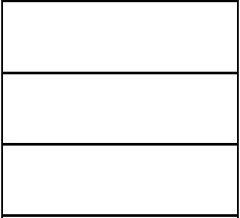# Rectangular 8136

Divide a square garden with a perimeter of 124 m into two rectangular gardens so that the fence of one garden is 10 m longer than the fence of the other. What dimensions will these rectangular gardens be?

a =  31
b =  18
c =  13
d =  31

### Step-by-step explanation:

a = 124/4
b+c = a
d = a
2(a+b) = 10 + 2(c+d)

a = 124/4
b+c = a
d = a
2·(a+b) = 10 + 2·(c+d)

4a = 124
a-b-c = 0
a-d = 0
2a+2b-2c-2d = 10

Row 2 - 1/4 · Row 1 → Row 2
4a = 124
-b-c = -31
a-d = 0
2a+2b-2c-2d = 10

Row 3 - 1/4 · Row 1 → Row 3
4a = 124
-b-c = -31
-d = -31
2a+2b-2c-2d = 10

Row 4 - 2/4 · Row 1 → Row 4
4a = 124
-b-c = -31
-d = -31
2b-2c-2d = -52

Pivot: Row 2 ↔ Row 4
4a = 124
2b-2c-2d = -52
-d = -31
-b-c = -31

Row 4 - -1/2 · Row 2 → Row 4
4a = 124
2b-2c-2d = -52
-d = -31
-2c-d = -57

Pivot: Row 3 ↔ Row 4
4a = 124
2b-2c-2d = -52
-2c-d = -57
-d = -31

d = -31/-1 = 31
c = -57+d/-2 = -57+31/-2 = 13
b = -52+2c+2d/2 = -52+2 · 13+2 · 31/2 = 18
a = 124/4 = 31

a = 31
b = 18
c = 13
d = 31

Our linear equations calculator calculates it.Did you find an error or inaccuracy? Feel free to write us. Thank you!

Tips for related online calculators
Do you have a linear equation or system of equations and looking for its solution? Or do you have a quadratic equation?

#### Grade of the word problem:

We encourage you to watch this tutorial video on this math problem: